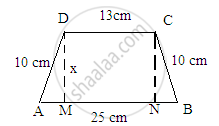Share

# The Parallel Sides of a Trapezium Are 25 Cm and 13 Cm; Its Nonparallel Sides Are Equal, Each Being 10 Cm, Find the Area of the Trapezium. - Mathematics

Course
ConceptArea of Trapezium

#### Question

The parallel sides of a trapezium are 25 cm and 13 cm; its nonparallel sides are equal, each being 10 cm, find the area of the trapezium.

#### Solution

Given:
The parallel sides of a trapezium are 25 cm and 13 cm.
Its nonparallel sides are equal in length and each is equal to 10 cm.
A rough sketch for the given trapezium is given below:In above figure, we observe that both the right angle trangles AMD and BNC are congruent triangles.
AD = BC = 10 cm
D  = CN = x cm
$\angle DMA = \angle CNB = 90^\circ$
Hence, the third side of both the triangles will also be equal.
$\therefore AM=BN$
Also, MN=13
Since AB = AM+MN+NB:
$\therefore 25=AM+13+BN$
$AM+BN=25-13=12 cm$
$Or, BN+BN=12 cm (\text{ Because AM=BN })$
$2 BN=12$
$BN=\frac{12}{2}=6 cm$
∴ AM = BN = 6 cm.
Now, to find the value of x, we will use the Pythagoras theorem in the right angle triangle AMD, whose sides are 10, 6 and x.
${(\text{ Hypotenuse })}^2 {=(\text{ Base })}^2 {+(\text{ Altitude })}^2$
${(10)}^2 {=(6)}^2 {+(x)}^2$
${100=36+x}^2$
$x^2 =100-36=64$
$x=\sqrt{64}=8 cm$
∴ Distance between the parallel sides = 8 cm
∴ Area of trapezium$=\frac{1}{2}\times( \text{ Sum of parallel sides })\times(\text{ Distance between parallel sides })$
$=\frac{1}{2}\times(25+13)\times(8)$
${=152 cm}^2$

Is there an error in this question or solution?

#### APPEARS IN

RD Sharma Solution for Mathematics for Class 8 by R D Sharma (2019-2020 Session) (2017 to Current)
Chapter 20: Mensuration - I (Area of a Trapezium and a Polygon)
Ex. 20.2 | Q: 16 | Page no. 24

#### Video TutorialsVIEW ALL 

Solution The Parallel Sides of a Trapezium Are 25 Cm and 13 Cm; Its Nonparallel Sides Are Equal, Each Being 10 Cm, Find the Area of the Trapezium. Concept: Area of Trapezium.
S